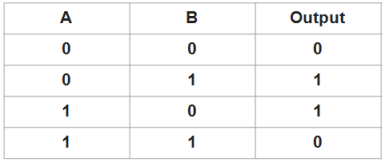# Find the number which occurs odd number of times

In an array having positive integers, except for one number which occurs odd number of times, all other numbers occur even number of times. Find the number.
Example -
Input : 2 5 9 1 5 1 8 2 8
Output: 9

Input : 2 3 4 3 1 4 5 1 4 2 5
Output: 4

## Algorithm/Insights

Algorithm 1:
Count the occurrence of each array element and check if it is odd or not. Print the element which has odd occurrence.
This algorithm uses two loops and runs in O(n2) time.

Algorithm 2:
Create a hash map to store the element as key and count of the element as value.
Iterate over the array elements. If the array element is not present in the array, then add the element to the map with count = 1. If the array element is already present because of a previous iteration, then update the count of the array by incrementing the count by 1.
Finally iterate over the map and print the element which has odd occurrence.
This algorithm takes O(n) time and O(n) auxiliary space.

Algorithm 3:
1. Initialize result = input.
2. Iterate over the array from i = 1 to length of the array and XOR the result with each element of the input array.
3. Once iteration over the array is done, print result as the output.

XOR operation
Truth Table -Truth Table of A XOR B shows that it outputs true whenever the input differs.

XOR operation of a number with itself results in 0.
Example:
Consider 5 XOR 5
Binary representation for 5 is 101. Hence applying bitwise XOR on 5 following rules given in the above truth table -
101 XOR 101 = 000 = 0
Now, if XOR is applied on the above result with 5, we get 5 XOR 5 XOR 5 as -
000 XOR 101 = 101 = 5

XOR operation on a number with itself even number of times will result in 0.
XOR operation on a number with itself odd number of times will result in the number itself.
This algorithm takes O(n) time and O(1) auxiliary space.

Code for all the above algorithms is given in Code Snippet section.

## Code Snippet

```
package com.ideserve.questions.saurabh;

import java.util.HashMap;
import java.util.Map;
import java.util.Map.Entry;

/**
* <b>IDeserve <br>
*
* @author Saurabh
*/
public class NumberOccuringOddNumberOfTimes {

public static void printNumberOccuringOddNumberOfTimes(int[] input) {
if(input == null || input.length == 0) {
System.out.println("Empty input array.");
return;
}
int result = input;
for (int i = 1; i < input.length; i++) {
result = result ^ input[i];
}
System.out.println("Number occurring odd number of times is " + result);
}

public static void printNumberOccuringOddNumberOfTimesHash(int[] input) {
if(input == null || input.length == 0) {
System.out.println("Empty input array.");
return;
}

Map<Integer, Integer> map = new HashMap<>();
for (int i = 0; i < input.length; i++) {
if(map.containsKey(input[i])) {
map.put(input[i], map.get(input[i]) + 1);
} else {
map.put(input[i], 1);
}
}

for(Entry<Integer, Integer> entry : map.entrySet()) {
if(entry.getValue() % 2 == 1) {
System.out.println("Number occurring odd number of times is " + entry.getKey());
return;
}
}
}

public static void printNumberOccuringOddNumberOfTimesNaive(int[] input) {
if(input == null || input.length == 0) {
System.out.println("Empty input array.");
return;
}

for(int i = 0; i < input.length; i++) {
int count = 1;
for (int j = 0; j < input.length; j++) {
if(input[i] == input[j] && i != j) {
count++;
}
}
if(count % 2 == 1) {
System.out.println("Number occurring odd number of times is " + input[i]);
break;
}
}
}

public static void main(String[] args) {
int[] input = {2, 3, 4, 3, 1, 4, 5, 1, 4, 2, 5};
printNumberOccuringOddNumberOfTimes(input);
}
}
```